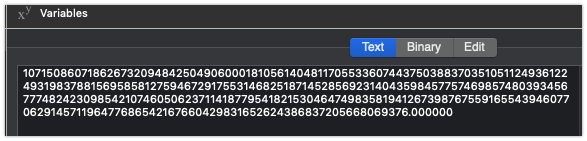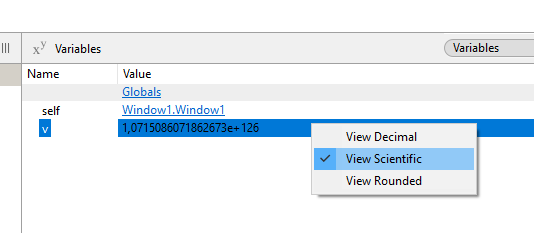# Maximum value of a double

According to the LR the maximum value of a double is:

Numerical limits

• The maximum value of a Double is: ±1.79769313486231570814527423731704357e+308
• The minimum value towards zero is: ±4.94065645841246544176568792868221372e-324

So, what am I doing wrong in this example since what I get is lower that the maximum?

Translated with DeepL

Hi Ramon,
any number can be written in exponential notation,
if for example we consider the following power of 2:
2^10 = 2 * 2 * 2 * 2 * 2 * 2 * 2 * 2 * 2 * 2 = 1024 = 1.024 * 10^3 = 1.024e+3
all of these writings are equivalent.

Now: 2^1000 = 2 * 2 * 2 * 2 … times 1000 … =10715086071862673165704665181841200401631588885282586599765471017463822740753974333291729869547101590312202274201558077931520 = (that’s 127 digits) = 1.0715086e+126

so the two writings 2^1000 and 1.0715086e+126 are equivalent,
they are two different ways to represent the same number.

Regards

Thank you Domenico,

But if I use this code:
Var v As Double = 1.79769313486231570814527423731704357 * 10^308
Break
MessageBox v.ToString

at the break I get the same value.
I have not checked what is the result of the MessageBox, so perhaps the problem is only in the Break and what the debugger window shows.

Hi Ramon,
typically numbers are represented in computer memory in exponential notation, so if you define it in exponential notation, in the debugger window you see it in the same exponential notation.
In the above example 2^1000 is not written in exponential notation but as a power of base 2 and exponent 1000.
So it’s converted to exponential notation, and that’s what the debugger window shows.

If I do 1.79…*10^308, I get the correct value (and increasing it gives an inf value). If I do 2^1000, I get the correct answer of 1.07e+126. Are you saying you get 1.07e+126 for both cases?

Mmmmh no.
2^1000 is actually 10715086071862673209484250490600018105614048117055336074437503883703510511249361224931983788156958581275946729175531468251871452856923140435984577574698574803934567774824230985421074605062371141877954182153046474983581941267398767559165543946077062914571196477686542167660429831652624386837205668069376
That’s 302 digits: 1.071509e+301

@Ramon_SASTRE Which version of Xojo are you using? Running on Windows/Mac/Linux?
Are you running in 64bits?

Yes. I am running in 64 bits.

It seems that there is a problem with huge numbers.
I’ve tried
`Var v As Double = 5e300/3`
and in the debugger I see
`1.6666666666666668e+126`
curiously again +126

Exactly.
I see the same value

SORRY. IT IS NOT THE SAME VALUE.
IT IS 1.7976931348623157e+126

Wow. Another bug. I think it’s Double.ToString as that doesn’T format correctly long doubles.

64639 - Double.ToString broken for big numebrs

There may be more issues, but since ToString is fairly new, it may be buggy.

That is the result I got from Xojo, running the latest version.
I also checked with online sources that it is correct

Thanks Christian. I think you are completely right.

1 Like

@Jeremie_L
2^1000 is actually … 1.071509e+301

Hi Jeremie,
I confirm that the value you indicated is the correct one, and also the string you get from
` MessageBox(v.ToSting)`
is correct.
But the debugger window still shows e+126

I am running the latest version of Xojo (2021r1.1) in 64-bit on Windows10.
I don’t understand.
Regards

If you make conversions of (int/double) datatypes including Double you’d want to use CType perhaps

``````Var v As Double = CType(2.0 ^ 1000.0, Double) // <- check the result in the debugger
Var s As String = v.ToString // <- check the result in the debugger
Break
``````

by the way the value of “v” in the debugger is a Xojo based conversion to string, out of your hands. Make sure you debug values you can show as you expect them to be so Var v As String above is giving you the v.ToString value, you can also try to show it as v.ToString(“0.000000000000000000000000000”) something like that.

Here are the results I got using the MBS plugins:

2^1000 using Doubles

1.071508607186267E+301

using BigNumberMBS

1.07150860718626732094842504906000181056140481170553360744375038837035105112489e+301

using BiggerNumberMBS

1.0715086071862673209484250490600018105614048117055336074437503883703510511249361224931983788156958581275946729175531468251871452856923140435984577574698574803934567774824230985421074605062371141877954182153046474983581941267398767559165543946077062914571196477686542167660429831652624386837205668069376e+301

I do not see the 1,07e+126 issue on macOS.

It seems like this is a problem with the debugger on Windows only.

We have a few issues here. After some tests I found:

Problem 1
Debugger: Windows shows e+126 for any double with 127 positions or more. Mac shows doubles with 127 digits.
Test code:

``````Dim a1 As Double = 5e127
Dim a2 As Double = 5e129
Dim a3 As Double = 5e135
Dim a4 As Double = 5e200
Dim a5 As Double = 5e300
Break
``````

Debugger shows:

Problem 2
Val(String) only uses the first 127 positions
Test code:

``````Dim s As String = "5000000000000000375872434582591043137357145321762041067414545511788829626212076023322705505488790177141329775194262631633387520"
Dim a1 As Double = Val(s)
Dim a2 As Double = Val(s + "00")
Dim a3 As Double = Val(s + "0000000000000000000000000000000000000000000000000000000000000000000")
Break
``````

Debugger shows:

Interesting that Double.ToString is working correctly using 2^1000 and the BiggerNumberMBS result above:
Test code

``````dim test As Double = 2^1000
dim test_f As String = format(test, "#.###############E")
dim test_s As String = test.ToString
Break
``````

Debugger shows:

I guess “test” on Windows will show e+126
if we click the pencil for test_s we see:and that is the exact same number as the BiggerNumberMBS result above:

What I think is happening:

• IDE debugger showing a limited double but has the correct information internally. Showing e+126 in Windows and limiting to 127 digits in mac
• Val() can’t handle strings with more than 127 characters, everything past that is converted to the same 127 digit double (not tested with all combinations)
• ToString is working correctly
1 Like

Good test Alberto.
Thank you.

2 Likes

Right now I don’t have access to a Windows 10 machine but running a VM with Virtualbox I get this:

I don’t know why you get e+126 and I don’t. Is there a setting somewhere? Could it be different using a real PC?

Hi Alberto,
In the debugger right-click on the value of interest and from the contextual menu that opens click on ‘View Scientific’.
Regards3 Likes
Forum for Xojo Programming Language and IDE. Copyright © 2021 Xojo, Inc.